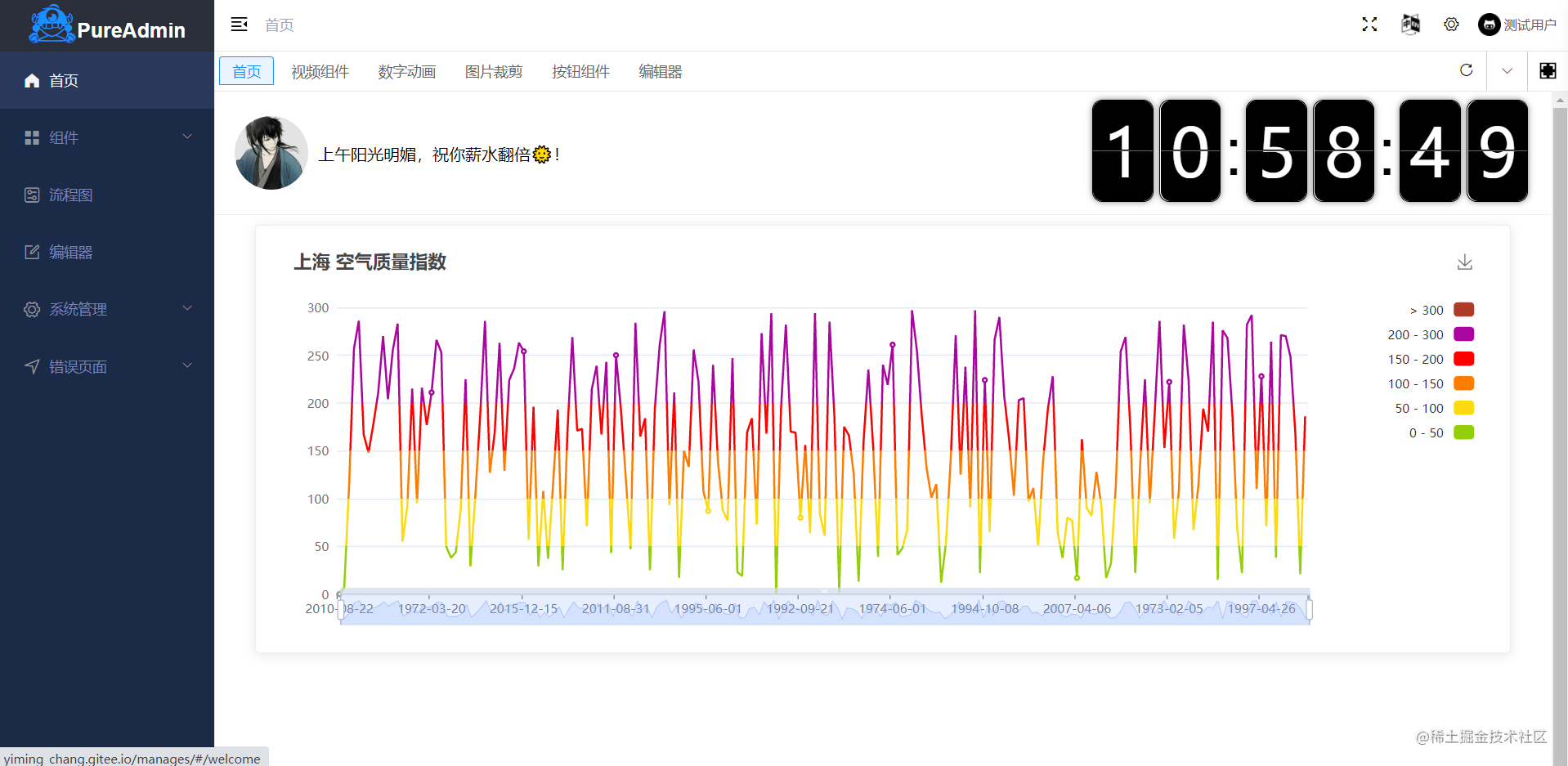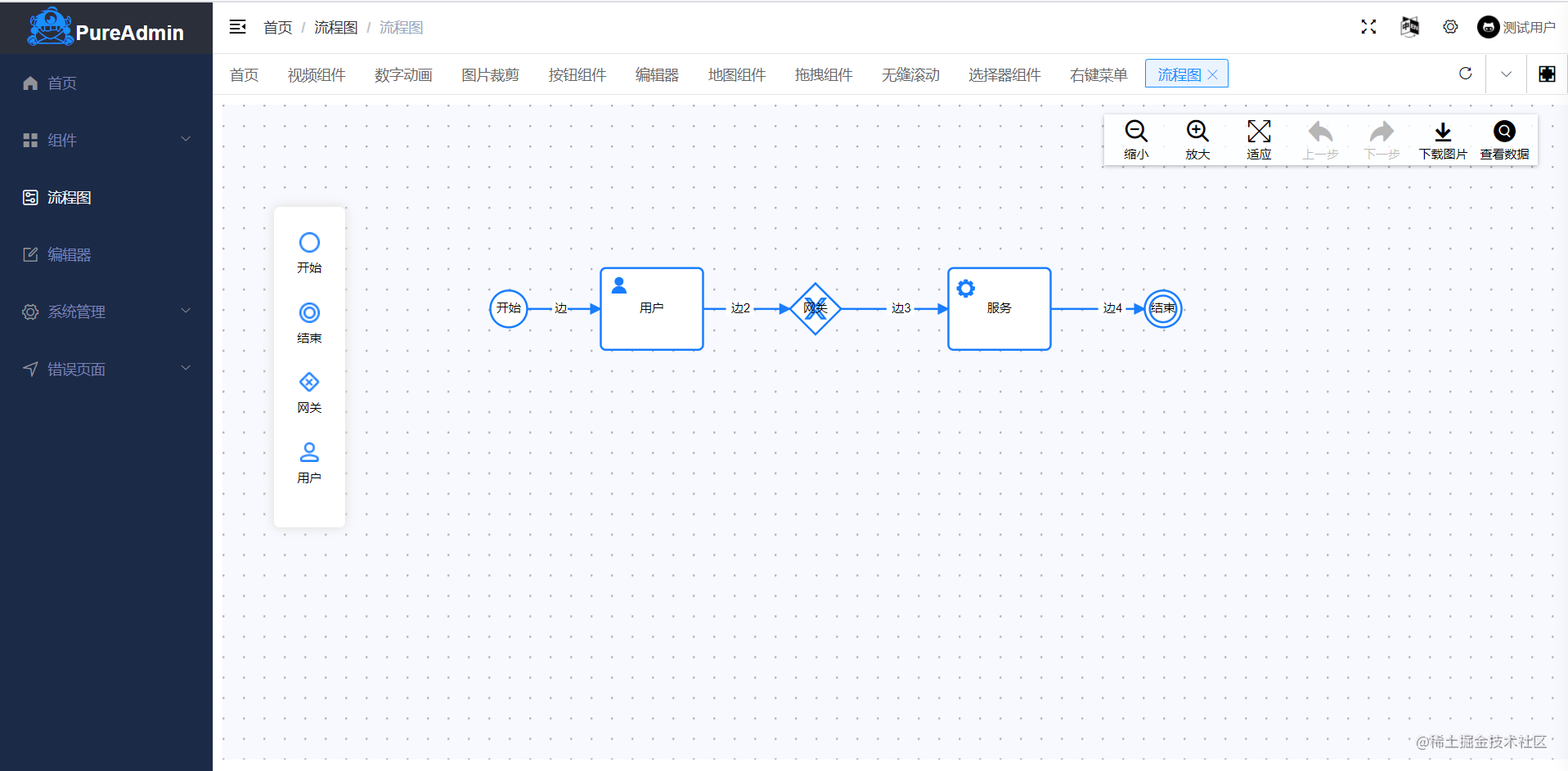# 如何让你的代码更优雅## 注释

### 如何使用注释？（在哪些地方需要添加注释）

通用的规则是在代码不清晰时需要添加注释，个人觉得包含以下几个方面：

1. 难于理解的代码添加注释（逻辑比较复杂，嵌套层级比较多，传递参数不明显等）

2. 可能被误认为错误的代码（没有按照正常套路出牌的代码 　　3. 数据的状态（就拿订单状态来说，订单可能有多钟状态，此时在写这段的叶落逻辑的时候，就把不同的状态表示的含义写上去，以后的维护，或者是有新人接收这个项目的时候能够更快的上手） 　　4. 其他注释（文档注释，变量注释，函数注释）

### 2.变量注释：

``````// difference
let uName = 'Symbol卢'; // 虽然用了驼峰，但是简写严重。无法直观看出变量的含义
// grace
let userName = 'Symbol卢'; // 语义化命名，不能过于缩写

### 3.函数的注释

``````/**
* @description: 数据类型的检测的第二种方式
* @param {any} data 要检测数据类型的变量
* @return {string} type 返回具体的类型名称【小写】
*/
export const \$typeOf = (data) => {
return Object.prototype.toString.call(data).replace(/\[object (\w+)\]/, '\$1').toLowerCase()
}

## 变量

### 1. let和const 取代 var

#### 定义常量

``````// difference
var myLove = 'My love is Code';//没有使用const进行命名，且常量名称没有全部大写

// grace
const MY_LOVE = 'My love is Code';

``````//要注意。静态字符串一律使用单引号或反引号（模板字符串），不使用双引号，动态字符串使用反引号，拒绝使用 + 进行字符串的拼接
// difference
const name = "Symbol卢";
const say = 'My name is' + name + 'and I love FE';

// grace
const NAME = 'Symbol卢';
const b = `My name is \${ NAME } and I love FE`;

#### 定义变量

``````if (true) {
let x = 'hello';
}
let name = () => {
// ...
}

``````// difference
one.js
window.name = 'Symbol卢';
two.js
window.name = 'Jack李';
three.js
window.name = 'Leo';

#### 给常量赋值

``````let lastName = fullName; //如果fullName=[],那么fullName=undefined 很容易就出问题了
let propertyValue = Object.attr; //如果Object={},那么Object.attr=undefined

``````let lastName = fullName || ''
let propertyValue=Object.attr || 0

（1）要按强类型风格写代码定义变量的时候要指明类型，并且在变量声明之后，不要随意的去更改变量的数据类型，改着改着搞不好就出问题了

``````// 假设声明三个变量a,b,c
let a,b,c; // difference，定义变量时没有指明类型
let a = "", b = [], c = {}; // good

// difference
let a = "";
...
a = 100; // 改变了定义变量时的类型(容易出错问题哦！)

let b = 5;
...
b = 200; // grace 定义了数据类型之后，就不要去随便的更改它

2、避免使用 `==` 进行逻辑判断（不要给自己的代码埋雷）

`==` 表示只要值相等即为真，`=== ` 要求不仅值相等，而且也要求类型相同，这句话相信大家也都知道

``````0  == ''          // true
0  == '0'         // true
'' == 0           // true
'' == '0'         // false
false == '0'        // true
false == 'false'    // false
false == undefined  // false
false == null       // false
null == undefined   // true
true == 1           // true

``````let total = "6";
if(total == 6) {} // difference
if(parseInt(total) === 6){} // grace 手动转换一下数据类型

## 数组

``````// 数组拷贝
// difference
let items=['1','2','3'];
let items2=['4','5','6'];
for (i = 0; i < items.lenght; i++){
items.push(items2[i]);
}

// grace
const itemsCopy = [...items];

// 扩展运算符简写
// joining arrays
const odd = [1, 3, 5];
const nums = [2 ,4 , 6].concat(odd);
// cloning arrays
const arr = [1, 2, 3, 4];
const arr2 = arr.slice()

// joining arrays
const odd = [1, 3, 5 ];
const nums = [2 ,4 , 6, ...odd];
console.log(nums); // [ 2, 4, 6, 1, 3, 5 ]
// cloning arrays
const arr = [1, 2, 3, 4];
const arr2 = [...arr];

• 使用数组成员对变量赋值时，优先使用解构赋值
``````const Arr = [1, 2, 3, 4];

// difference
const first = Arr;
const second = Arr;

// grace
const [first, second] = Arr; // 有简单的方法。肯定要用简单的方法啦

## 函数

### 函数的命名

``````let isEmpty = () => {...}
let canCreateDocuments = () => {...}
let hasLicense = () => {...}

// 不用「否定」语法命名函数
//difference
let isNotSupport = () => {};
let canNotUpdate = () => {};

// grace
let isSupport = () => {};
let canUpdate = () => {};

``````let sendEmailToUser = (user) => {
....
}
let geUserInfo = (user) => {
....
}
let setUserInfo = (user) => {
....
}

### 优先使用箭头函数

``````let arr [18, 19, 20, 21, 22,23,24,25]
// commonly
function findAge (arr, age) {
return arr.filter(function (num) {
return num === age
})
}
// grace 是不是看着更简介优雅了
let findAge = (arr, age)=> arr.filter(num => num === age)

### 函数的入参

``````// difference
function getImages(api, true, false); // true和false啥意思，没有个注释的话，看上去就是一脸懵逼
// grace
function getImages({
imageApi: api,
includePageBackground: true, // 一目了然，知道这些true和false是啥意思
compress: false,
})

### 接收参数

``````// 假设现在的场景是获取用户的信息上的现用名，和曾用名
// difference
function getFullName(user) {
const firstName = user.firstName;
const lastName = user.lastName;
}

// commonly
function getFullName(obj) {
const { firstName, lastName } = obj;
}

// grace
function getFullName({ firstName, lastName }) {
}

// grace 给它个默认值
function getFullName({firstName, lastName = '无'}) {
}

// 觉得参数的名称太长，咱再来个重命名  解构时重命名简化命名
// grace
function getFullName ({firstName: first, lastName: last}) {

}

### 参数校验

``````function test(name, sex = 1) {
if (!name){ // 不满足条件尽早抛出错误
throw new Error('没有传递name参数');
}
// ...
}

### 函数的出参

``````// 注意，返回参数的数据类型要是固定的，
// difference返回了不同的数据类型
function getResult(cont) {
if(count < 0) {
return "";
} else {
return count * 10;
}
}

// grace
function getResult(cont) {
if(count < 0) {
return -1;
} else {
return count * 10;
}
}

// 函数返回多个值，推荐使用对象作为函数的返回值
// commonly
function processInput(input) {
return [left, right, top, bottom];
}

// grace
function processInput(input) {
return { left, right, top, bottom };
}
const { left, right } = processInput(input);

### 立即执行函数

``````(() => {
console.log('感觉这篇文章还可以的话，欢迎点赞+关注+转发');
})();

### 优先使用函数式编程

``````// difference
for(i = 1; i <= 10; i++) {
a[i] = a[i] +1;
}
// grace
let b = a.map(item => ++item) //是不是更简洁了

### 函数中过多的采用if else ..

``````// commonly
if (a === 1) {
//...
} else if (a ===2) {
// ...
} else if (a === 3) {
//...
} else {
//...
}

// 一般
switch(a) {
case 1:
//....
case 2:
//....
case 3:
//....
default:
//....
}

// grace ===》》》 Object
const fruit = {
1: ['1', '11'],
2: ['2', '22'],
3: ['3', '33']
};
let test = (a) => {
return fruit[a] || [];
}

// grace ===》》》 Map
const fruit = newMap()
.set('张三', ['张三丰', '张三力'])
.set('李四', ['李思维', '李素丽'])
let test = (a) => {
return fruit.get(a) || [];
}

// grace ===》》》filter
const fruits = [
{ name: '张三', work: 'js' },
{ name: '李四', work: 'php' },
{ name: '王五', work: 'java' },
];
let test = (a) => {
return fruits.filter(item => item.name === a);
}

// grace===》》》策略模式
let handler = {
1: () => {
//....
},
2: () => {
//....
},
3: () => {
//....
},
default: () => {
//....
}
}
handler[a]() || handler['default']()

## 其他

#### 在if中判断数组长度的判断

``````// difference
if (arr.length !== 0) {
//...
}

// grace
if (arr.length) {
//...
}

// difference
if (arr.length === 0) {
//...
}

// grace
if (!arr.length) {
//...
}

### 逻辑运算符

``````if (a === 1) {
b()
}
//可以写成
a === 1 && b()

const arr = [1,2,3];
if(!arr.length){
b()
}
//可以写出
arr.length || b()

// &&判断依赖的键是否存在，防止报错'xxx of undfined'
let user = {
name: 'Symbol卢',
age: 18,
children: {
name: '小Symbol卢'
}
}
let childrenName = user.children && user.childre.name

#### 能使用三目运算符（三元运算符）就使用，留着舍不得用，要留着过年用嘛

``````// difference
const a = '';
let b;
if( a === '' ){
b = 'no'
} else {
b = 'ok'
}

const a = ''
let b = a ? 'no' : 'ok'; // 'ok'

``````/**
* @description: 数据类型的检测的第二种方式
* @param {any} data 要检测数据类型的变量
* @return {string} type 返回具体的类型名称【小写】
*/
export const isTypeOf = (data) => {
return Object.prototype.toString.call(data).replace(/\[object (\w+)\]/, '\$1').toLowerCase()
}

#### 对于for循环优先使用命令式编程

``````// difference
let result=[]
let numberArr=[1,2,3,4,5,6]
for(i = 1; i <= 10; i++) {
if(numberArr[i]>4){
result.push(numberArr[i])
}
}

#### 使用 Array.includes 来处理多重 || 条件

``````// difference
if (a === 1 || a === 2 || a === 3 || a === 4) {
//...
}
// grace
let arr = [1, 2, 3, 4]
if (arr.includes(a)) {
//...
}

``````// grace
const users = [
{ name: '张三', sex:1 },
{ name: '李四', sex:2 },
{ name: '王五', sex:1 }
];
function test() {
// 条件：（简短形式）所有的用户都必须是女
const isAllGirl = users.every(item => item.sex === 1);

// 条件：至少一个用户是男的
const isAnyMan = users.some(item => item.sex === 2);

}

#### 使用正则表达式

``````const imgType ='jpg'
if(imgType === 'jpg' || imgType === 'png' || imgType === 'gif'){
console.log('is image')
}

// 使用match匹配正则表达式
const imgType='jpg'
if(imgType.match(/.*?(gif|png|jpg)/gi)){
console.log('is image')
}

#### 连接字符串

``````let name = 'Symbol'
let message = 'Hello,I\'m' + name + 'take care '// 采用传统加号，看着很冗余,且容易出错
// 艾玛，模板字符香，真想
let message = `Hello,I'm \${name} take care `

## 总结